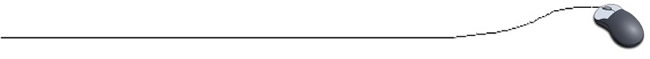Demo Program - Working with Arrays

import java.util.Scanner;

public class Demo1
{
public static void main(String[] args)
{
final int sizeOfArray = 25;
int [ ] grades = new int [sizeOfArray];
int total = 0;

// Fill the array
for (int i = 0; i < sizeOfArray; i++)
{
}

// Print the array
for (int i = 0; i < sizeOfArray; i++)
{
}

 What is the advantage of using a CONSTANT (final) to represent the size of the array? Answer By using a CONSTANT, only one line will need to be changed to allow this program to work with arrays of differing sizes.

 What is the purpose of the counter total ? Answer To add the grades as they are entered. Getting ready to do the average.

 What is the purpose of using a cast to (double) for finding the average? Answer To avoid integer division from occurring.
// Find the average

double average = (double) total/sizeOfArray;
System.out.println("\nAverage of grades is " + average + ".");
}
}